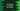# How to compare two strings in C sharp# How to compare two strings in C sharp :

In this tutorial, we will learn how to compare two strings in C#. We will write one program to take two strings as input from the user. It will then compare the strings and print out the result i.e. if the first string is greater, smaller or equal to the second string. With this tutorial, you will learn how to compare two strings, how to read user inputs and how to use if-else conditions in C#.

## Algorithm :

We will use the below algorithm :

1. Ask the user to enter the first string. Read and store it in a variable.

2. Ask the user to enter the second string. Read and store it in a different variable.

3. Compare both strings.

4. Print the message to the user accordingly.

## string.Compare(string,string) method :

We are going to use string.compare method that takes two strings and returns one integer. The first argument is the_ first string_ and the second argument is the second string to compare. The return integer value indicates the relative position of the strings in sort order or the lexical relationship between the strings.

• If the result is less than 0, the first string precedes the second string in the sorting order.

• If the result is 0, both strings will be in the same position of sort order or both strings are equal.

• If the result is greater than 0, the first string follows the second string in the sort order.

## C# program :

``````using System;

namespace dotnet_sample
{
class Program
{
static void Main(string[] args)
{
//1
string str1, str2;

//2
Console.WriteLine("Enter the first string : ");

//3
Console.WriteLine("Enter the second string : ");

//4
if (string.Compare(str1,str2) == 0)
{
Console.WriteLine("Both strings are equal");
}
else if(string.Compare(str1, str2) < 0)
{
//5
Console.WriteLine("The first string is smaller than the second string");
}
else
{
//6
Console.WriteLine("The first string is greater than the second string");
}
}
}
}
``````

### Explanation :

The commented numbers in the above program denote the step numbers below :

1. Create two string variables _str1 _and str2.

2. Ask the user to enter the first string. Read it and store it in the str1 variable.

3. Similarly, read the second string and store it in the str2 variable.

4. Now using one_ if-else if-else_ condition to compare these strings. The first if is checking if both strings are equal or not using Compare method. If both are equal, print that to the user.

5. In the _else if _ block, check if the result of Compare is less than zero or not. If yes, that means the first string precedes the second string in sort order or the first string is smaller than the third string.

6. If both 4 and 5 checks are failed, the first string should be greater than the second string or the result of Compare should be greater than 0.

### Sample Output :

``````Enter the first string :
hello
Enter the second string :
hello
Both strings are equal

Enter the first string :
hello
Enter the second string :
world
The first string is smaller than the second string

Enter the first string :
world
Enter the second string :
hello
The first string is greater than the second string
``````

### Conclusion :

In this tutorial, we have learned how to compare two strings in C sharp. Try to run the example shown above and drop one comment below if you have any queries.